## Example Questions

### Example Question #1 : How To Find Out If Lines Are Perpendicular

what would be the slope of a line perpendicular to

4x+3y = 6

3/4

4/3

-3/4

-4/3

4

3/4

Explanation:

switch 4x+ 3y = 6 to "y=mx+b" form

3y= -4x + 6

y = -4/3 x + 2

m = -4/3; the perpendicular line will have the negative reciprocal of this line so it would be 3/4

### Example Question #2 : How To Find Out If Lines Are Perpendicular

Which line is perpendicular to the line between the points (22,24) and (31,4)?

y = x

y = –3x + 5

the line between the points (9, 5) and (48, 19)

the line between the points (4, 7) and (7, 4)

y = .45x + 10

y = .45x + 10

Explanation:

The line will be perpendicular if the slope is the negative reciprocal.

First we need to find the slope of our line between points (22,24) and (31,4). Slope = rise/run = (24 – 4)/(22 – 31) = 20/–9 = –2.22.

The negative reciprocal of this must be a positive fraction, so we can eliminate y = –3+ 5 (because the slope is negative).

The negative reciprocal of –2.22, and therefore the slope of the perpendicular line, will be –1/–2.22 = .45, so we can also eliminate y = x (slope of 1).

Now let's look at the line between points (9, 5) and (48, 19). This slope = (5 – 19)/(9 – 48) = .358, which is incorrect.

The next answer choice is y = .45x + 10. The slope is .45, which is what we're looking for so this is the correct answer.

To double check, the last answer choice is the line between (4, 7) and (7, 4). This slope = (7 – 4) / (4 – 7) = –1, which is also incorrect.

### Example Question #1 : Perpendicular Lines

Which best describes the relationship between the lines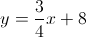and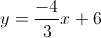?

The equations describe the same line.

The lines are perpendicular.

None of the above.

The lines are parallel.

The lines are perpendicular.

Explanation:

We first need to recall the following relationships:

Lines with the same slope and same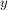-intercept are really the same line.

Lines with the same slope and different-intercepts are parallel.

Lines with slopes that are negative reciprocals are perpendicular.

Then we identify the slopes of the two lines by comparing the equations to the slope-intercept form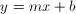, whereis the slope andis the-intercept. By inspection we see the lines have slopes of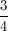and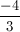. Since these are different, the "parallel" and "same line" choices are eliminated. To test if the slopes are negative reciprocals, we take one of the slopes, change its sign, and flip it upside-down. Starting withand changing the sign gives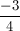, then flipping gives. This is the same as the slope of the second line, so the two slopes are negative reciprocals and the lines are perpendicular.

### Example Question #4 : How To Find Out If Lines Are Perpendicular

Which of the following lines is perpendicular to the line defined as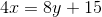?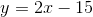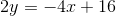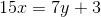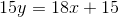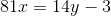Explanation:

To begin, the best thing to do is to put your equation into slope-intercept format. That is, into the format:For your equation, you need to solve for: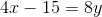, which is the same as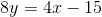Then, divide both sides by: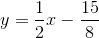So, the slope of this line is.  The perpendicular of a line is opposite and reciprocal. Therefore, the perpendicular line will have a slope of. Of the options given, onlymatches this (which you can figure out when you solve for).

### Example Question #5 : How To Find Out If Lines Are Perpendicular

Which of the following lines is perpendicular to the line passing through the points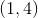and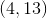?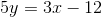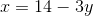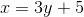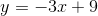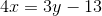Explanation:

Remember, to be perpendicular, two lines must have opposite and reciprocal slopes. Therefore, you need to begin by solving for the slope of your given line. You do this by finding: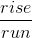For two points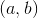and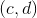, this is: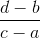For our points, this is: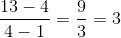The slope of the perpendicular line will be (remember) opposite and reciprocal. Therefore, it will be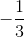.  Now, among your equations, the only one that has this slope is:If you solve this for, you get: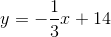According to the slope-intercept form (), this means that the slope is.

### Example Question #1 : How To Find Out If Lines Are Perpendicular

Which of the following lines is perpendicular to the line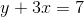?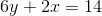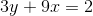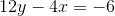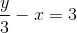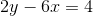Explanation:

Perpendicular lines will have slopes that are negative reciprocals of one another. Our first step will be to find the slope of the given line by putting the equation into slope-intercept form.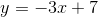The slope of this line is. The negative reciprocal will be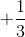, which will be the slope of the perpendicular line.

Now we need to find the answer choice with this slope by converting to slope-intercept form.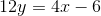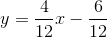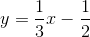This equation has a slope of, and must be our answer.

### Example Question #2 : How To Find Out If Lines Are Perpendicular

Which of the following lines is perpindicular to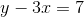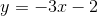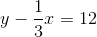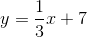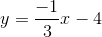Explanation:

When determining if a two lines are perpindicular, we are only concerned about their slopes. Consider the basic equation of a line,, where m is the slope of the line. Two lines are perpindicular to each other if one slope is the negative and reciprocal of the other.

The first step of this problem is to get it into the form,, which is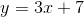. Now we know that the slope, m, is. The reciprocal of that is, and the negative of that is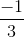. Therefore, any line that has a slope ofwill be perpindicular to the original line.

### Example Question #1 : How To Find Out If Lines Are Perpendicular

Which of the following equations represents a line that is perpendicular to the line with points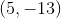and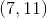?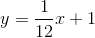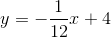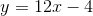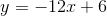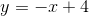Explanation:

If lines are perpendicular, then their slopes will be negative reciprocals.

First, we need to find the slope of the given line.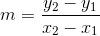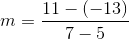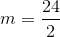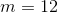Because we know that our given line's slope is, the slope of the line perpendicular to it must be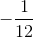.

Tired of practice problems?

Try live online GRE Math prep today.